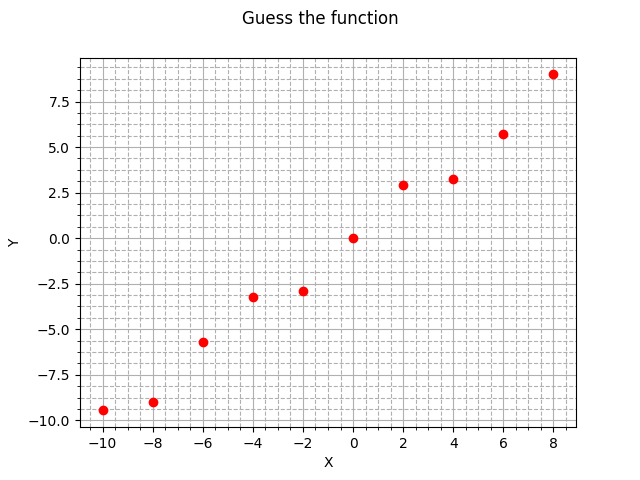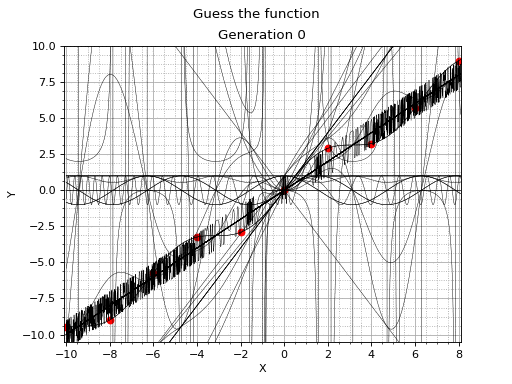{{ message }}

# Symbolic regressionSymbolic regression is a type of regression analysis that searches the space of mathematical expressions to find the model that best fits a given dataset.

No particular model is provided as a starting point to the algorithm. Instead, initial expressions are formed by randomly combining mathematical building blocks such as operators, analytic functions, constants and state variables.

# Genetic programming

While Genetic Programming (GP) can be used to perform a wide variety of tasks, symbolic regression is probably one of the most frequent area of application (the term symbolic regression stems from earlier work by John Koza on GP).

GP builds a population of simple random formulae to represent relationships among independent variables in order to predict new data. Successive generations of formulae (aka individuals / programs) are evolved from the previous one by selecting the fittest individuals from the population to undergo genetic operations.

The fitness function that drives the evolution can take into account not only error metrics (to ensure the models accurately predict the data), but also special complexity measures, thus ensuring that the resulting models reveal the data's underlying structure in a way that's understandable from a human perspective. This facilitates reasoning and favors the odds of getting insights about the data-generating system.

# The code

```#include "kernel/vita.h"

int main()
{
// DATA SAMPLE
// (the target function is `x + sin(x)`)
std::istringstream training(R"(
-9.456,-10.0
-8.989, -8.0
-5.721, -6.0
-3.243, -4.0
-2.909, -2.0
0.000,  0.0
2.909,  2.0
3.243,  4.0
5.721,  6.0
8.989,  8.0
)");

vita::src_problem prob(training);

// SETTING UP SYMBOLS
prob.insert<vita::real::sin>();
prob.insert<vita::real::cos>();
prob.insert<vita::real::sub>();
prob.insert<vita::real::div>();
prob.insert<vita::real::mul>();

// SEARCH & RESULT
vita::src_search s(prob);
const auto result(s.run());

std::cout << "\nCANDIDATE SOLUTION\n"
<< vita::out::c_language << result.best.solution
<< "\n\nFITNESS\n" << result.best.score.fitness << '\n';
}```

(for your ease the above code is in the examples/symbolic_regression.cc file)

All the classes and functions are placed into the vita `namespace`.

## Line by line description

`#include "kernel/vita.h"`

`vita.h` is the only header file you must include: it's enough for genetic programming (both symbolic regression and classification), genetic algorithms and differential evolution.

Data points, in the `f(X), X` format, are stored in the `training` input stream (in general they're in a CSV file):

```  std::istringstream training(R"(
-9.456,-10.0
-8.989, -8.0
...
)");```

Points come from an unknown target function `f`. The discovery of this function is our goal.

On a graph:`vita::src_problem prob(training);`

The `src_problem` object contains everything needed for evolution: parameters, dataset... Here we use the constructor that initilizes almost all the sensible values and the training set.

...almost all, something remains to be done. Input variables are automatically inserted reading the input data. The remaining building blocks for individuals / formulae / programs have to be specified:

```  prob.insert<vita::real::sin>();
prob.insert<vita::real::cos>();
prob.insert<vita::real::sub>();
prob.insert<vita::real::div>();
prob.insert<vita::real::mul>();```

Vita comes with batteries included: `vita::real::sin`, `vita::real::cos`, `vita::real::add` are part of a predefined primitive set.

Now all that's left is to start the search:

```vita::src_search s(prob);
const auto result(s.run());```

and print the results:

```std::cout << "\nCANDIDATE SOLUTION\n"
<< vita::out::c_language << result.best.solution
<< "\n\nFITNESS\n" << result.best.score.fitness << '\n';```

`vita::out::c_language` is a manipulator that make it possible to control the output format. `python_language` and `mql_language` are other possibilities (see individual.h for the full list).

What you get is something like:

``````[INFO] Reading dataset from input stream...
[INFO] ...dataset read. Examples: 10, categories: 1, features: 1, classes: 0
[INFO] Number of layers set to 1
[INFO] Population size set to 72
Run 0.     0 (  1%): fitness (-55.793)
Run 0.     0 (  2%): fitness (-15.4303)
Run 0.     0 ( 95%): fitness (-8.39641e-06)
Run 0.     9 (  2%): fitness (-8.39641e-06)
[INFO] Elapsed time: 0.074s
[INFO] Training fitness: (-8.39641e-06)

CANDIDATE SOLUTION
(X1+(X1+sin(X1)))-X1

FITNESS
(-8.39641e-06)
``````

Graphically this is what happen to the population:(if you're curious about the animation take a look at examples/symbolic_regression02.cc and examples/symbolic_regression02.py)

### GO ON ON →